Rounding Numbers to Thousands in Microsoft Excel 2010

In this article we will learn how we can round the numbers to thousands in Microsoft Excel 2010.

To round the numbers in thousands we can use Custom Format and Round function in Microsoft Excel 2010 and 2013.

We can round the numbers in Thousands from the 2 different ways.

1st Custom Format

2nd Round Function
Custom Format:- We can use Custom Number Format, to display a Number in thousands or Display it in some Color. It’s a tool from in old versions of excel when there was no Conditional Formatting option in Excel. All conditional options are performed by using Custom Number Format.

To round the number to thousands we will use the Custom Format.

Let’s take an example and understand how we can round the numbers to thousands.

We have categorized Income data. In which column D contains City Name, column E contains Gender and column F contains Income in \$. We want to format income in thousands.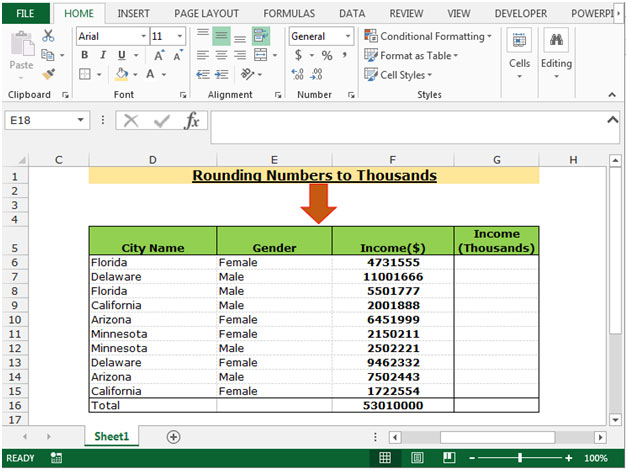To Round the income in thousands follow below given steps:-

• Select the range F6:F16 and copy by pressing the key Ctrl+C and paste in the range G6:G16 by pressing the key Ctrl+V on your keyboard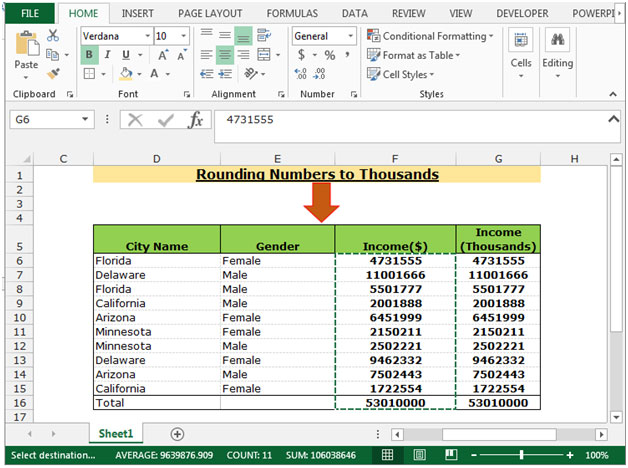• Select the range G6:G16 and press the key Ctrl+1 on your keyboard.
• Format Cells dialog box will appear.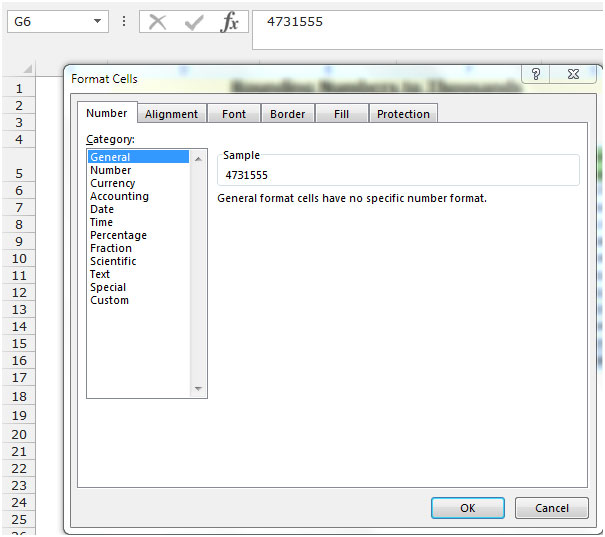• In the Number Tab, Click on Custom.
• After clicking on the Custom, related options will get appear.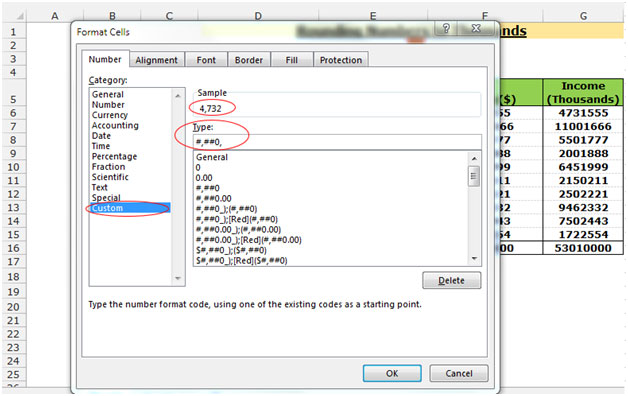• In the type box write the format to round the number in thousands #,##0,
• The function will return the rounded figure in thousands.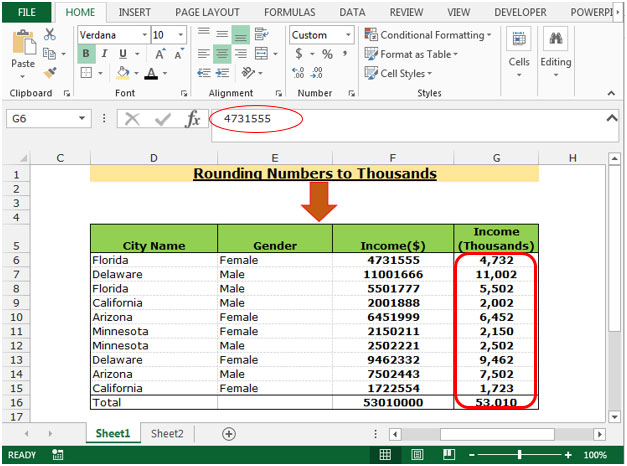Note: - When we add the total sometimes it may not appear to add up. In the above example, if we have 10 cells with amounts of 5301000 and total them, the total will show 53010. This total appears to be wrong.
You can use Round function to ignore this problem.

ROUND: - This function is used to round a number to a specified number of digits.

Syntax of “ROUND” function:       =ROUND (number, num_digits)

Example: Cell A1 contains the number 456.25

=ROUND(A1, 0), function will return 456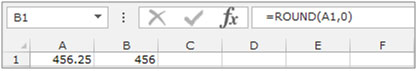Let’s understand with a simple exercise. Insert a column in the salary data.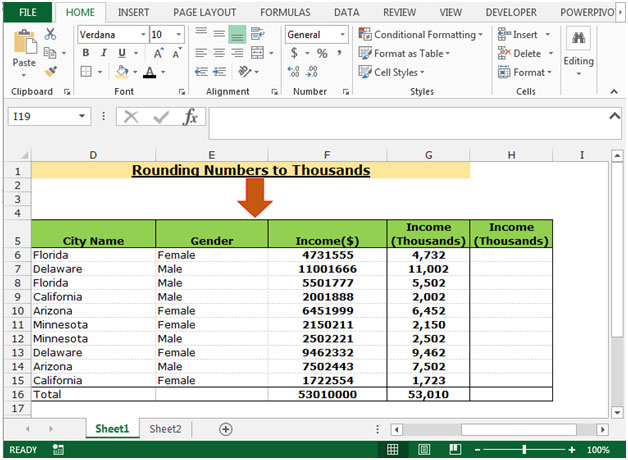• Select the Cell H6 and write the formula.
• =ROUND(F7,-3), press enter.
• The function will round the number in thousands.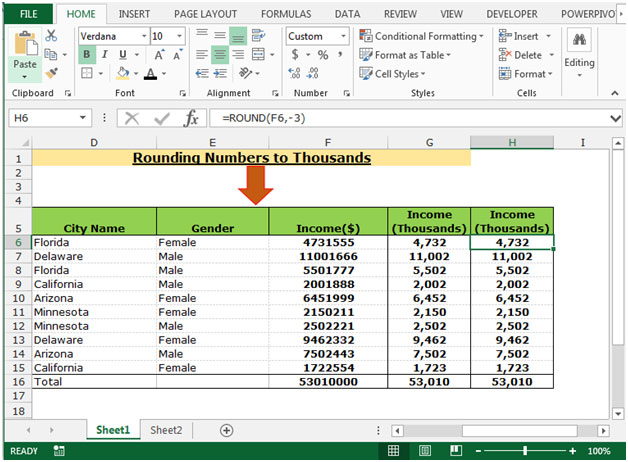This is the way we can round the number in thousands by using the formula and Custom Format in Microsoft Excel.

1."I thought

=ROUND(A1,-3)

would do the trick"

2."My version of this tip is:

A1=13546,67

round(A1/1000;0)*1000 will yield 13000 as a result."

3."INT(ROUND(A1,0))

e.g 1000.987654 is changed to 1001

(Whoops!)"

4."INT(ROUND(A1*1000,0))/1000

e.g 1000.987654 is changed to 1000.988

=INT(ROUND(A1,0))

e.g 1000.987654 is changed to 1000.1"

5.I use the "comma" style which comes with excel by default. It reads _(* #,##0.00_);_(* (#,##0.00);_(* "-"??_);_(@_) and if you replace the two .00 with a comma , it would provide you with numbers rounded to the nearest thousand.

6.I believe this format only changes the way the data is displayed. True rounding changes the value stored in the cell.

7.I have been using the following formula: #,###,;[Red](#,###,);- ; This seems to work. (PS leaving out the - ; at the end will return a blank cell when a zero is entered.)

8.After the mask is typed in and I click on OK, an entry in the sell of 11,321 produces "0 (11)" sans the quotes, with the entry in red. A check of the mask finds it in the following format: "[Red]##,#0_;(#,##0,);- ;." Me thinks more information is needed

9.I followed the instructions as they're described here into the "type" box, but it doesn't appear down with the other formats and I'm not able to select it. And I missing something?

Terms and Conditions of use

The applications/code on this site are distributed as is and without warranties or liability. In no event shall the owner of the copyrights, or the authors of the applications/code be liable for any loss of profit, any problems or any damage resulting from the use or evaluation of the applications/code.